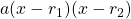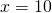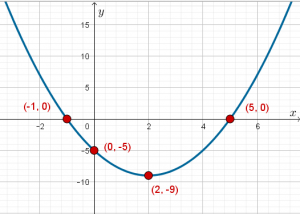# Three forms of a Quadratic Expression

A quadratic expression has three common presentations.

1. Standard (or polynomial) form:2. Factored form3. Completed square (or vertex) formLet’s see the expressionin these three forms:

1. Standard form is simply2. Factored form3. Completed square formThese three forms are equivalent. No matter what value ofyou might choose, the three forms will compute to the same value.

Let’s check with1.2.3.Now, spot checking like this is not a proof. I could have engineered that 10 would work, yet nothing else does. Beginning with one form, and algebraically changing its form will constitute a proof. We’ll do that work at the end of this page.

Let’s see the graph of this function,.Notice that theinterceptis the constant in the standard form.

Notice that theintercepts seem to be related to the factored form,.

Notice that the vertex (turning point) seems to be related to the completed square form.

In this unit, we find out how to algebraically convert from one form to another, and find out what each form is particularly useful for.

# Proof of Equivalence

Show thatShow thatAs Euclid postulated a long time ago, things that are equal are equal to each other. So ifandthenTherefore all three expressions are equivalent.